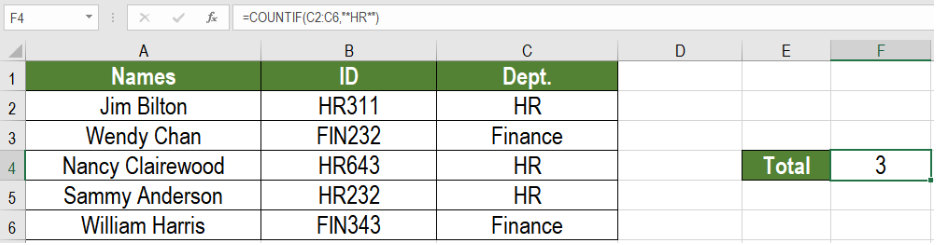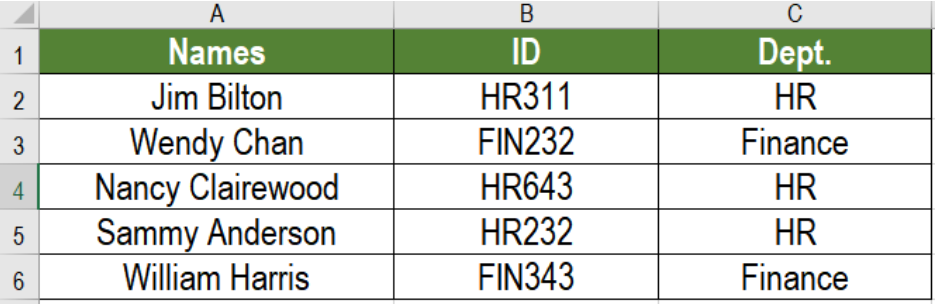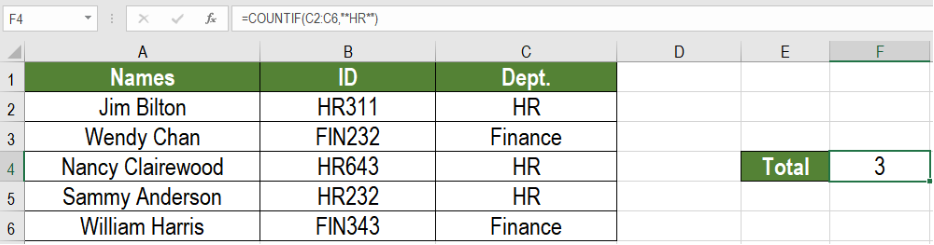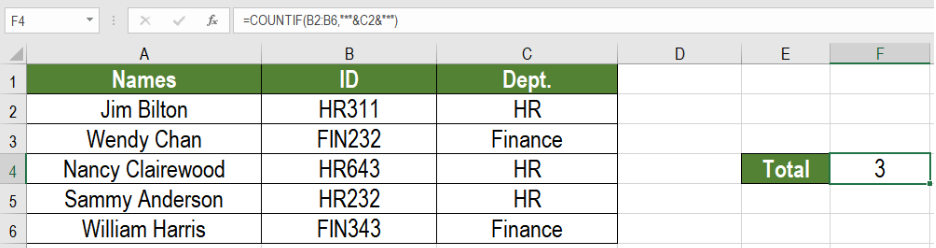Get instant live expert help with Excel or Google Sheets“My Excelchat expert helped me in less than 20 minutes, saving me what would have been 5 hours of work!”

#### Post your problem and you’ll get Expert help in seconds.

Your message must be at least 40 characters
Our professional Expert are available now. Your privacy is guaranteed.

# How to Count Cells that Contain Specific Text

Analyzing large chunks of data often requires to count cells containing specific text. Excel allows us to do it in a simple way. We can use the COUNTIF function to count cells that contain specific text. In this tutorial, we will learn how to count cells that contain specific text.Figure 1. Example of Counting Cells that Contain Specific Text

## Generic Formula

`=COUNTIF(range,"*text*")`

• Range: This value is required. It is the range of cells where we want to count the text.
• Text: This value is also required. It is the specific text that we want to cout. Here, the asterisk sign (“*”) represents a wildcard that matches any number of characters.

## How the Formula Works

Here, COUNTIF counts the total cells containing the provided text in the range. It matches the content of the cells against the wildcard pattern. This wildcard matches any number of characters. Thus, itl counts all cells containing the text in any position. Excel returns the total number of time the text arrives in the range.

## Setting Up Data

The following example uses an employee information data set. Column A, B and C has the employee names, ID and departments.Figure 2. The Sample Data

To find the total number of employees from the HR department:

• Go to cell F4.
• Assign the formula` =COUNTIF(C2:C6,"*HR*") `to cell F4.
• Press Enter.Figure 3. Applying the Formula to Count Cells Containing Specific Text

This will show the number of employees in the HR department in cell F4.

## Notes

We can change the previous formula to use the contents of another cell as the text criteria. To find the number of employees in the HR department using the ID’s, we need to use the formula:

`=COUNTIF(B2:B6,"*"&C2&"*")`Figure 4. Using the Wildcard to Count Cells that Contain Specific Text

This shows the same result as before using the text HR from the cell C2 to look into the ID’s that matches the text HR.

Most of the time, the problem you will need to solve will be more complex than a simple application of a formula or function. If you want to save hours of research and frustration, try our live Excelchat service! Our Excel Experts are available 24/7 to answer any Excel question you may have. We guarantee a connection within 30 seconds and a customized solution within 20 minutes.

### Did this post not answer your question? Get a solution from connecting with the expert.Another blog reader asked this question today on Excelchat:
Solution examplesI need a formula to count the number of rows that contain at least one of two possible phrases, but not give me the total number of instances that both phrases occur overall.
Solved by E. B. in 33 minsi have a table with Dates, First names , Last names and i want to count how many times a name occurs, but if a name occurs more than once on a particular date i need to only count it once. this is on excel and not google sheets
Solved by F. H. in 31 minsNeed a formula that counts (1,2,3,4 ect..in cell M21) a range of cells, N4:N20, which are annual premium values. But I only want to add a sale count if the average monthly premium is above \$30 per sale. So if someone has 4 sales but they don't equal a total of \$120/mth (\$30 x 4 = \$120)... they would only get a count of 3 sales.
Solved by S. E. in 60 minsHello, I am trying to create a total color column? Can anyone help?
Solved by I. A. in 60 minsAny rep that has orders over 500 and occurrences at zero for each month, for a 6 month period. I need a sum
Solved by C. E. in 60 mins## Subscribe to Excelchat.coAnother blog reader asked this question today on Excelchat: